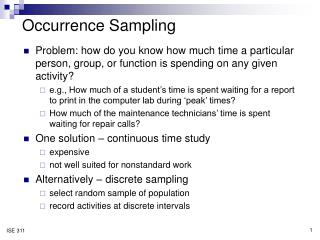DownloadDownload PresentationOccurrence Sampling

# Occurrence Sampling

Download Presentation## Occurrence Sampling

- - - - - - - - - - - - - - - - - - - - - - - - - - - E N D - - - - - - - - - - - - - - - - - - - - - - - - - - -
##### Presentation Transcript

1. Occurrence Sampling • Problem: how do you know how much time a particular person, group, or function is spending on any given activity? • e.g., How much of a student’s time is spent waiting for a report to print in the computer lab during ‘peak’ times? • How much of the maintenance technicians’ time is spent waiting for repair calls? • One solution – continuous time study • expensive • not well suited for nonstandard work • Alternatively – discrete sampling • select random sample of population • record activities at discrete intervals

2. Determining Sample Size • Law of diminishing returns • amount of information grows proportionately with the square root of sample size, n • cost of information grows directly with n • therefore, there will be a sample size beyond which additional information is not worth the cost of additional study • Sample size depends on … • desired absolute accuracy, A • note difference between absolute and relative accuracy, s • (estimated) proportion of occurrence, p • desired confidence level, c

3. Sample size example • It is estimated that students in the computer lab must wait in line for their document to print about 45% of the time. To justify an additional printer, you wish to verify that estimate within 15% (relative accuracy) and with a confidence level of 90%. • Solution, p = 0.4 A = (0.45)(0.15) = 0.0675 c = 90%  z = ± 1.64 -.0675 +.0675 table 10.1, pg. 137 0.45 0.3825 0.5175

4. Sampling – design and data collection • Overcoming the 3 problems in obtaining a representative sample: • Define reasonable strata (categories) for data collection • time of day (morning, afternoon, evening, etc.) • day of week (or weekend/weekday, week in the month, etc.) • gender • region • socio-economic status • level of education / training • etc. Base sample size on smallest estimated proportion • Randomness • defining random sampling times/locations • randomness with restrictions table 10.3, pg. 142 (ERGO, Excel)

5. Data Gathering • Who & how? • person or machine? • additional duty for employee or hire temp? • automated data collection? • level of detail • the problem of influence • does the presence of the observer affect the actions or performance of the entity being observed? • techniques to minimize influence • unobtrusive observation • random sample • distance, video, etc. • communication with the observed

6. Data Analysis & Use • Comparing frequency data • procedure on pg. 145 • Example: is there a difference in number of times there are students waiting for printouts between morning and afternoon? na = nb = 100

7. Frequency example • Solution, 1. Smallest of 4 numbers = 25 2. Other number in the column = 36 3. “Observed contrast” = 11 4. from Table 10.4, minimum contrast = ______ 5. Compare observed contrast Answer: Morning is / is not different from afternoon.

8. Data Analysis & Use • Other comparison methods • χ2 (independence) or t-test to test for significant difference in means • control charts to test for time (or sequence) effects • Purpose of the analysis – determine if data should remain stratified or can be combined • if no difference, combine data and refer to overall proportions • if there is a difference, keep data, analysis, and conclusions separate